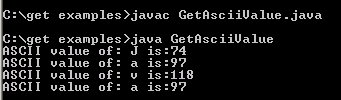# Get Ascii Value

In this example you will learn how to print ascii value of a character this is the the simplest way to change the type a character.### Get Ascii Value

In this example you will learn how to print ascii value of a character this is the the simplest way to change the type a character.# Get Ascii Value

To print ascii value of a character this is the the simplest way to change the type a character. Here in the example given below we will select character from a given string and change their type to integer that will be their ascii value.

Here is the video tutorial of "How to convert string to ASCII in Java?":

Method charAt() of String class is used to select character in the given string on basis of given index value as argument.

 public class GetAsciiValue { public static void main(String[] args) { String s = "Java"; for (int i=0; i

Output will be displayed as: